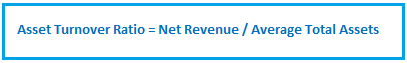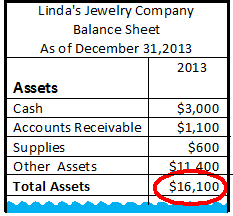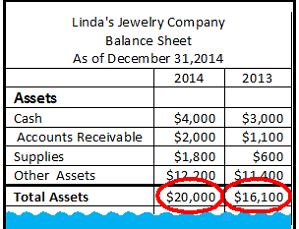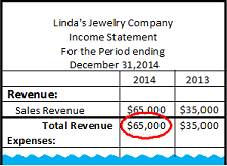# How to Calculate Asset Turnover Ratio: Formula & Example

Lesson Transcript
Instructor
Tina Van Rikxoord

Tina holds a PhD in Accounting, a Master of Business in Information Security Management, and a Master of Arts in History

Expert Contributor
Steven Scalia

Steven completed a Graduate Degree is Chartered Accountancy at Concordia University. He has performed as Teacher's Assistant and Assistant Lecturer in University.

The asset turnover ratio is one of the items that companies and potential stockholders look at in order to figure out how well a company's money is working for it. In this lesson, we will learn how to calculate a company's asset turnover ratio. Updated: 12/12/2019

## Asset Turnover Ratio Defined

Every business has assets, or things that the company owns and uses in its business in order to make money. These assets can include not just tangible items like cash, supplies, buildings, and equipment, but also intangible assets like trademarks and copyrights. The asset turnover ratio is a number that shows how much revenue is being earned for every dollar the company has spent on assets. It represents how well a company uses its assets to make money.

An error occurred trying to load this video.

Try refreshing the page, or contact customer support.

Coming up next: How to Calculate Current Yield: Definition, Formula & Graph

### You're on a roll. Keep up the good work!

Replay
Your next lesson will play in 10 seconds
• 0:00 Asset Turnover Ratio Defined
• 0:30 Formula
• 3:10 Interpreting Asset…
• 4:32 Lesson Summary
Save Save

Want to watch this again later?

Timeline
Autoplay
Autoplay
Speed Speed

## Formula

The formula for figuring the asset turnover ratio is:To see how to use this formula, let's look at the example of a company that makes jewelry. We'll call it Linda's Jewelry, and Linda is the owner. To make her jewelry Linda needs tools like beads, wire, string, glue, and work tables. She will also need computers and software to keep track of sales, inventory, and other administrative items. All of these items are considered to be assets.

Let's say the company just started in 2013 and had \$16,100 worth of total assets in its first year. Since the company has only been in business for one year, we can use the total assets listed on the balance sheet as the average total assets.Average Total Assets for 2013 = \$16,100

If the company has been in operation for at least two years, you will need to calculate the average of the total assets for the past two years. Let's say that in its second year of operation, Linda's Jewelry had \$20,000 in assets.To calculate the average total assets, add the total assets for the current year to the total assets for the previous year,and divide by two.

Average Total Assets for 2014 = (Assets for 2014 + Assets for 2013) / 2

Average Total Assets for 2014 = (\$20,000 + \$16,100) / 2

Average Total Assets for 2014 = \$18,050

Now that we have figured out the average total assets, we can use it in the formula.

The second piece of information that we need for the formula is the company's net revenue, which is the sales revenue after deducting various expenses. Net revenue is taken directly from the income statement. The net revenue used in the formula is generally called total revenue on the income statement. Let's say that in its first year Linda's Jewelry earns \$35,000 in net revenue. In the second year, Linda's Jewelry earns \$65,000 in net revenue.Now, let's say Linda wants to understand how well the assets the company has purchased are contributing to creating that income. She would calculate the company's asset turnover ratio to find out. So, for Linda's Jewelry:

Asset Turnover Ratio for 2013 = \$35,000 / \$16,100

Asset Turnover Ratio for 2013 = 2.14

Asset Turnover Ratio for 2014 = \$65,000 / \$18,050

Asset Turnover Ratio for 2014 = 3.60

To unlock this lesson you must be a Study.com Member.

## Calculation Of The Asset Turnover Ratio: A Business Case

You will be asked to compute the asset turnover ratio by using the formula provided in the Lesson and the information in the business case below. You will then be asked to interpret the ratio's meaning. The objective of this practice case is to assess your ability to (1) compute the asset turnover ratio and (2) interpret the ratio.

Somatel Foods is a company based in New York, NY. The company operates a small grocery store in a busy Manhattan neighborhood. Below is some selected information from its latest financial statements.

ItemCurrent year (\$)Prior year (\$)
Sales558,000498,000
Sales returns and allowances (Contra Revenue Account)23,00047,000
Total Assets354,000326,000

Requirement 1

Calculate the Asset turnover ratio for the current year using the information provided above. (Hint: Be sure to calculate Net Revenue first!)

Requirement 2

Somatel has hired consultants to assess its performance relative to its peers. Researchers found that the average grocery food store in Manhattan had an Asset Turnover Ratio of 2.01 in the current year. Using this information, comment on how Somatel Foods is performing relative to its competitors. Is Somatel Foods more effective at generating income from its assets?

## Solution:

Requirement 1

=Net revenue / Average assets

=(558,000-23,000)/((354,000+326,000)/2)

=1.57 times

Requirement 2

Somatel's asset turnover ratio is BELOW the industry average. This means that the company is less effective at generating income from its assets and thus should try to optimize its revenue cycle.

### Register to view this lesson

Are you a student or a teacher?